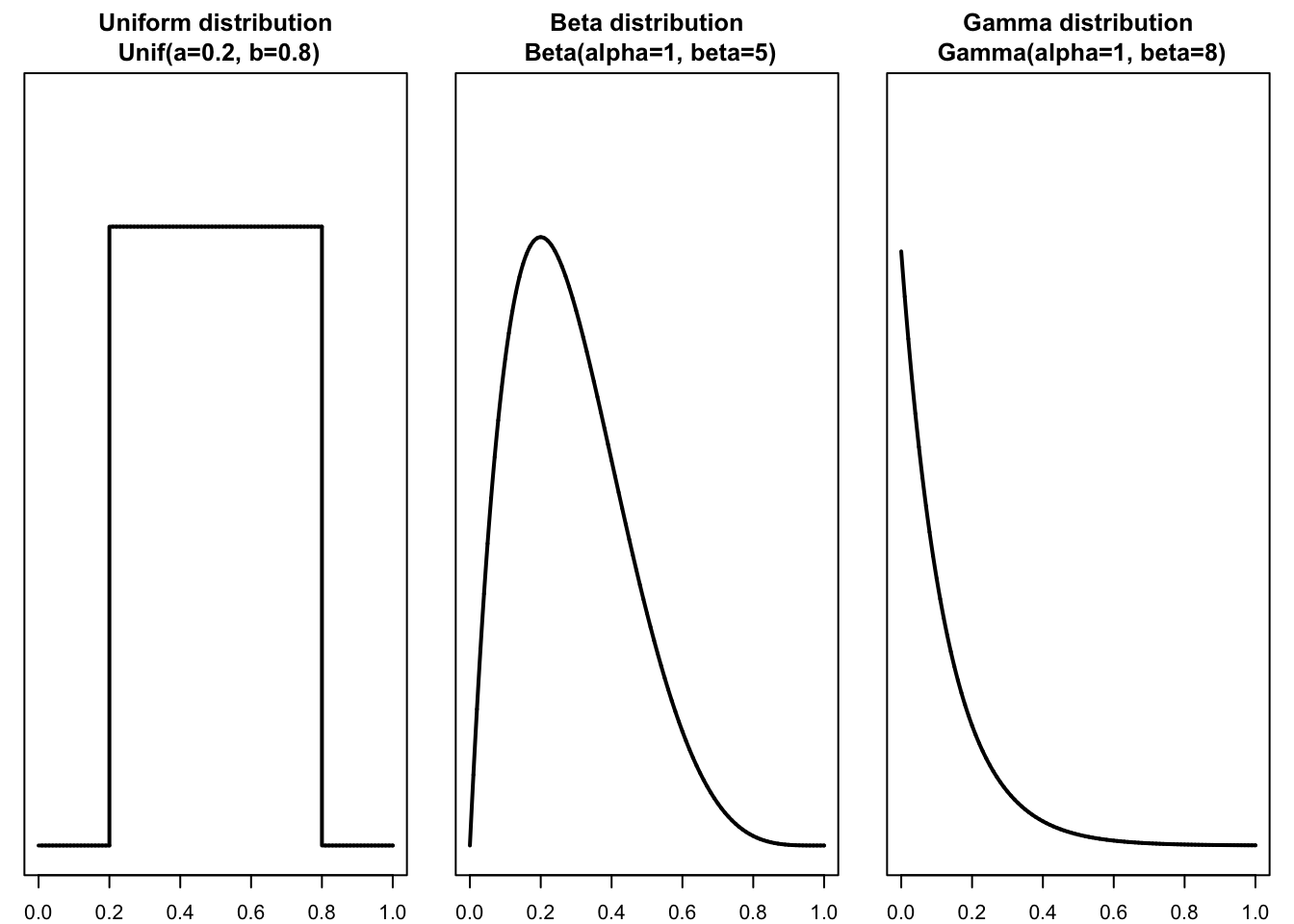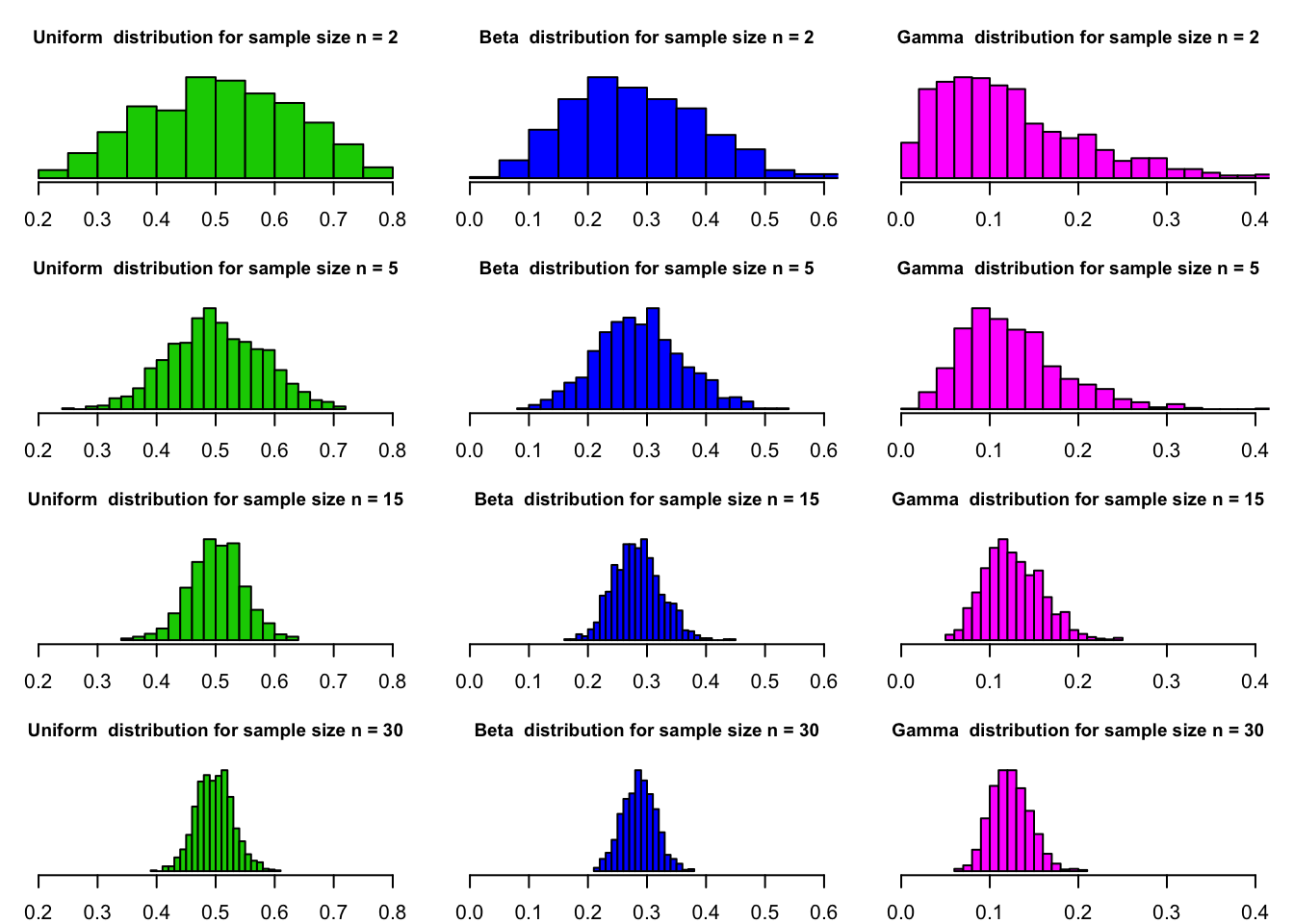#### Shape of the Sampling Distribution

The shape of the sampling distribution relates to the following two cases.
1. The population from which samples are drawn has a normal distribution.
2. The population from which samples are drawn does not have a normal distribution.

#### Sampling from a population which is not normally distributed

In the previous section we discussed the shape of sample distributions if sampling from a normally distributed population. However, in real life applications we often do not know the actual shape of the population distribution.

In order to understand, how the shape of the distribution of the population of interest affects the shape of the sampling distribution we conduct an experiment. Let us consider three different continuous probability density functions: the uniform distribution, the beta distribution, and the gamma distribution. We do not go into details here, however the figure below shows, that these three PDFs are not normally distributed.Now we conduct the same experiment as in the previous section. For a large enough number of times (trials = 1000) we sample from each particular distribution. However, this time each particular sample has a sample size $$n=2,5,15,30$$. For each sample we calculate the sample mean $$\bar x$$ and visualize the empirical probabilities after 1,000 trials.

trials <- 1000
n <- c(2,5,15,30) #sample size

# emtpy matrix to store results of computations
out.unif <- matrix(nrow = trials, ncol = length(n))
out.beta <- matrix(nrow = trials, ncol = length(n))
out.gamma <- matrix(nrow = trials, ncol = length(n))

# random sampling
for (i in seq(trials)){
for (j in seq(length(n))) {
out.unif[i,j] <- mean(runif(n[j], min = 0.2, max = 0.8))
out.beta[i,j] <- mean(rbeta(n[j], shape1 = 2, shape2 = 5))
out.gamma[i,j] <- mean(rgamma(n[j], shape = 1, rate = 8))
}
}

# plotting
#set plotting variables
par(mfrow = c(4,3), mar = c(2,1,3,1))
plotting.variables <- list(out.unif, out.beta, out.gamma)

#generate 12 histogram plots and store them in a list
h.list <- list(NULL)
h.list.pos <- 1
for (i in seq(1,length(n))){
for (j in seq(1,3)){
out <- plotting.variables[[j]]
h.list[[h.list.pos]] <- hist(out[,i],
breaks = 'Scott',
plot = FALSE)
h.list.pos <- h.list.pos+1
}
}

#loop through the list of histograms and plot them
xlim.list <- rep(list(c(0.2,0.8), c(0,0.6), c(0,0.4)),4)
n.list <- list(rep(n,3), rep(n,3), rep(n,3), rep(n,3))
n.vector <- c(rep(n,3), rep(n,3), rep(n,3), rep(n,3))
main.vector <- rep(c('Uniform ', 'Beta ', 'Gamma '),4)
color <- rep(c(3,4,6),4)

for (i in seq(1,length(h.list))){
plot(h.list[[i]],
freq = FALSE,
xlim = xlim.list[[i]],
ylab = '',
yaxt = 'n',
col = color[i],
main = paste(main.vector[i], 'distribution for sample size n =', n.vector[i]),
cex.main=0.9)
}The figure shows that, in case of a population that is not normally distributed, the sampling distributions are not normal, when $$n < 30$$. However, the sampling distributions approximate a normal distribution, when $$n > 30$$. Also notice that the spread of the sampling distribution decreases as the sample size increases.

According to the central limit theorem, for a large sample size $$(n > 30)$$, the sampling distribution is approximately normal, irrespective of the shape of the population distribution (Mann 2012).

The mean and standard deviation of the sampling distribution of $$\bar x$$ are, respectively,

$\mu_{\bar x} = \mu \qquad \text{and} \qquad \sigma_{\bar x}=\frac{\sigma}{\sqrt{n}}$

The sample size is usually considered to be large if $$n \ge 30$$.

Owing to the fact that the sampling distribution approximates a normal distribution, the area under the curve of a sampling distribution yields probabilistic information about sample statistic.

Recall the Empirical Rule, also known as the 68-95-99.7 rule. Consequently, applied to the sampling distribution the 68-95-99.7 rule implies that

• about 68.26% of the sample means will be within one standard deviation of the population mean,

• 95.44% of the sample means will be within two standard deviations of the population mean, and

• about 99.74% of the sample means will be within three standard deviations of the population mean.